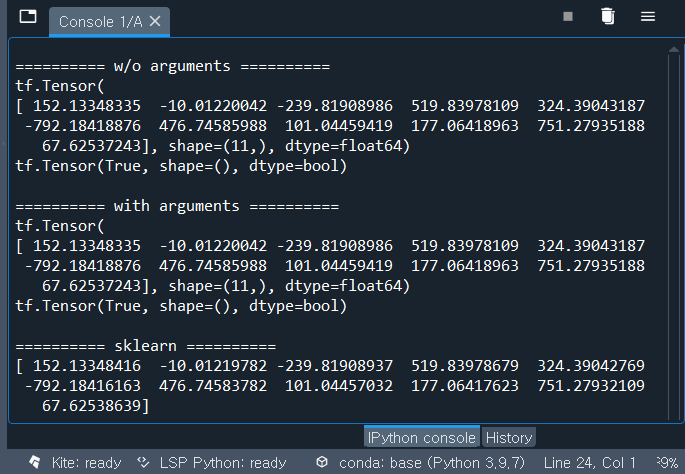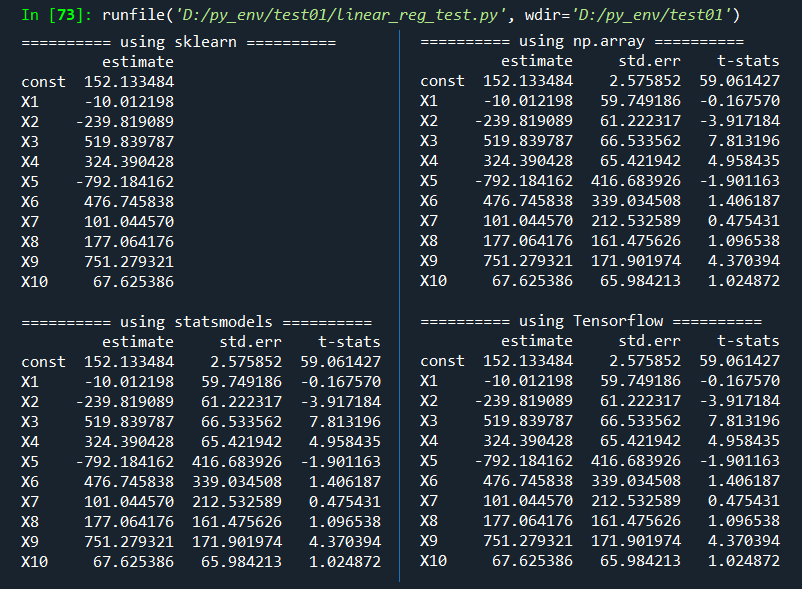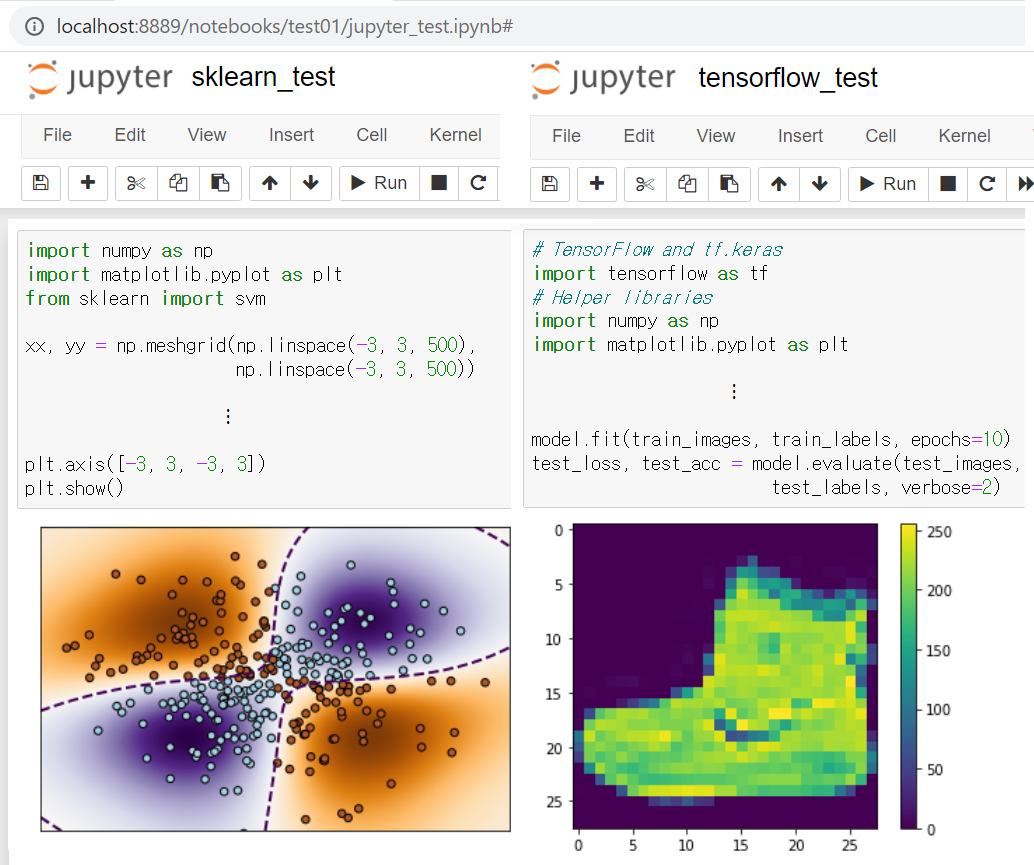# Articles by Sang-Heon Lee

### Python Troubleshooting Q&A

August 26, 2022 | Sang-Heon Lee

This is just a small collection of common error messages and solutions that we encounter when using Python. Python Troubleshooting Q&A 1. Pyfolio - AttributeError: 'numpy.int64' object has no attribute 'to_pydatetime' ...### Multiple Linear Regression using Tensorflow Optimization

March 15, 2022 | Sang-Heon Lee

This post implements the optimization based estimation of multiple linear regression model using Tensorflow. With this example, we can learn basic implementations of functions in Python and a numerical optimization in Tensorflow. Opti...### Multiple Linear Regression using Tensorflow

March 15, 2022 | Sang-Heon Lee

This post implements the standard matrix based estimation of multiple linear regression model using Tensorflow. With this example, we can learn some basic vector or matrix operations in Tensorflow and also Python. Linear Regression us...### Installing Python and Tensorflow with Jupyter Notebook Configurations

March 5, 2022 | Sang-Heon Lee

For a machine or deep learning modeling, Python is widely used with Tensorflow. This post explains the an installation of Python, Tensorflow and configuration of Jupyter notebook as a kickstart towards ML/DL modeling. Python, Tensor...Gradient Descent (GD) is the basic optimization algorithm for machine learning or deep learning. This post explains the basic concept of gradient descent with python code. Gradient Descent Parameter Learning Data is the outcome of action or activity. \begin{align} y, x \end{align} Our focus is to predict the ...